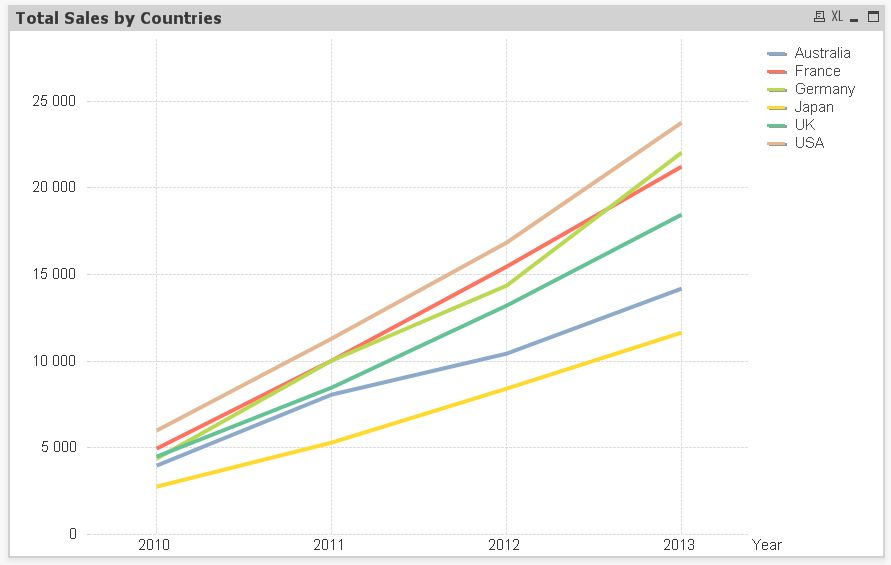# QlikView App Development

Discussion Board for collaboration related to QlikView App Development.

HighlightedNot applicable

## Accumulation sum in pivot table

Hello, I have a following data

Year, Country, Sales

2010, USA, 6013

2011, USA, 5295

2012, USA, 5551

2013, USA, 6932

2010, UK, 4512

2011, UK, 3976

2012, UK, 4691

2013, UK, 5276

2010, Japan, 2765

2011, Japan, 2567

2012, Japan, 3111

2013, Japan, 3234

2010, Germany, 4374

2011, Germany, 5673

2012, Germany, 4322

2013, Germany, 7654

2010, France, 4965

2011, France, 5097

2012, France, 5419

2013, France, 5732

2010, Australia, 3966

2011, Australia, 4087

2012, Australia, 2376

2013, Australia, 3784

];

If I create a new pivot with Year and Country dimensions

I can easily calculate:

Sales by Country  =Sum(Sales)

Total in country dimension =RangeSum(Above(Sum(Sales),0,RowNo()))

And overall Total =RangeSum(Above(TOTAL Sum(Sales), 0, RowNo(TOTAL)))

How can I calculate an overall total by countries? I want full accumulation sum only by countries through the years. This can be easily achieved by swapping dimensions, but this method does not suit me.

Tags (2)
1 Solution

Accepted SolutionsMVP

## Re: Accumulation sum in pivot table

Hi, before I copied the wrong expression, I wanted to to say this:

If(IsNull(Above(TOTAL Acc, Count(DISTINCT TOTAL Country))), 0 , Above(TOTAL Acc, Count(DISTINCT TOTAL Country)))+Sum(Sales)

Acc is the name of the expression

10 RepliesMVP

## Re: Accumulation sum in pivot table

Did you try straight chart for this it will give good performance.

Regards

Anand

Contributor III

## Re: Accumulation sum in pivot table

Hi Oleg,

not sure, if it fullfill your request...

RangeSum(Above(aggr(Sum(Sales), Country), 0, RowNo(TOTAL)))

BR

MNot applicable

## Re: Accumulation sum in pivot table

I want to get Line Chart like thisBut only way i see is to make an espression for each country.

=RangeSum(Above(Sum({<Country={'Australia'}>}Sales),0,RowNo()))

Contributor III

## Re: Accumulation sum in pivot table

In this case, you can use Year, Country as dimensions and sum(sales) as expression, but mark Full Accumulation.Not applicable

## Re: Accumulation sum in pivot table

Yes, it works.

But can i do it without built-in Accumulation?MVP

## Re: Accumulation sum in pivot table

Hi, before I copied the wrong expression, I wanted to to say this:

If(IsNull(Above(TOTAL Acc, Count(DISTINCT TOTAL Country))), 0 , Above(TOTAL Acc, Count(DISTINCT TOTAL Country)))+Sum(Sales)

Acc is the name of the expressionNot applicable

## Re: Accumulation sum in pivot table

It works! Now I will try to understand how it works ))). Do you think it is easiest way?MVP

## Re: Accumulation sum in pivot table

Probably there is a better and easier way, but I don't get how to do it right now.

Martin's solution is the easiest way if you can fit that in your requirements.

The expression I suggest will only work if there are the same number countries each year. It takes the last accumulated value 6 rows above (6=Number of countries) and sum the sales of the current row.

The first IsNull is to check if this is the first value for each country, and sum '0' instead of NULL (NULL+Value = NULL, 0+Value=Value)Partner

## Re: Accumulation sum in pivot table

Hi,

You can use the Alt() function like :

Alt(Above(TOTAL Acc, Count(DISTINCT TOTAL Country)), 0) + Sum(Sales)

Alt : The alt function returns the first of the parameters that has a valid number representation.

Best